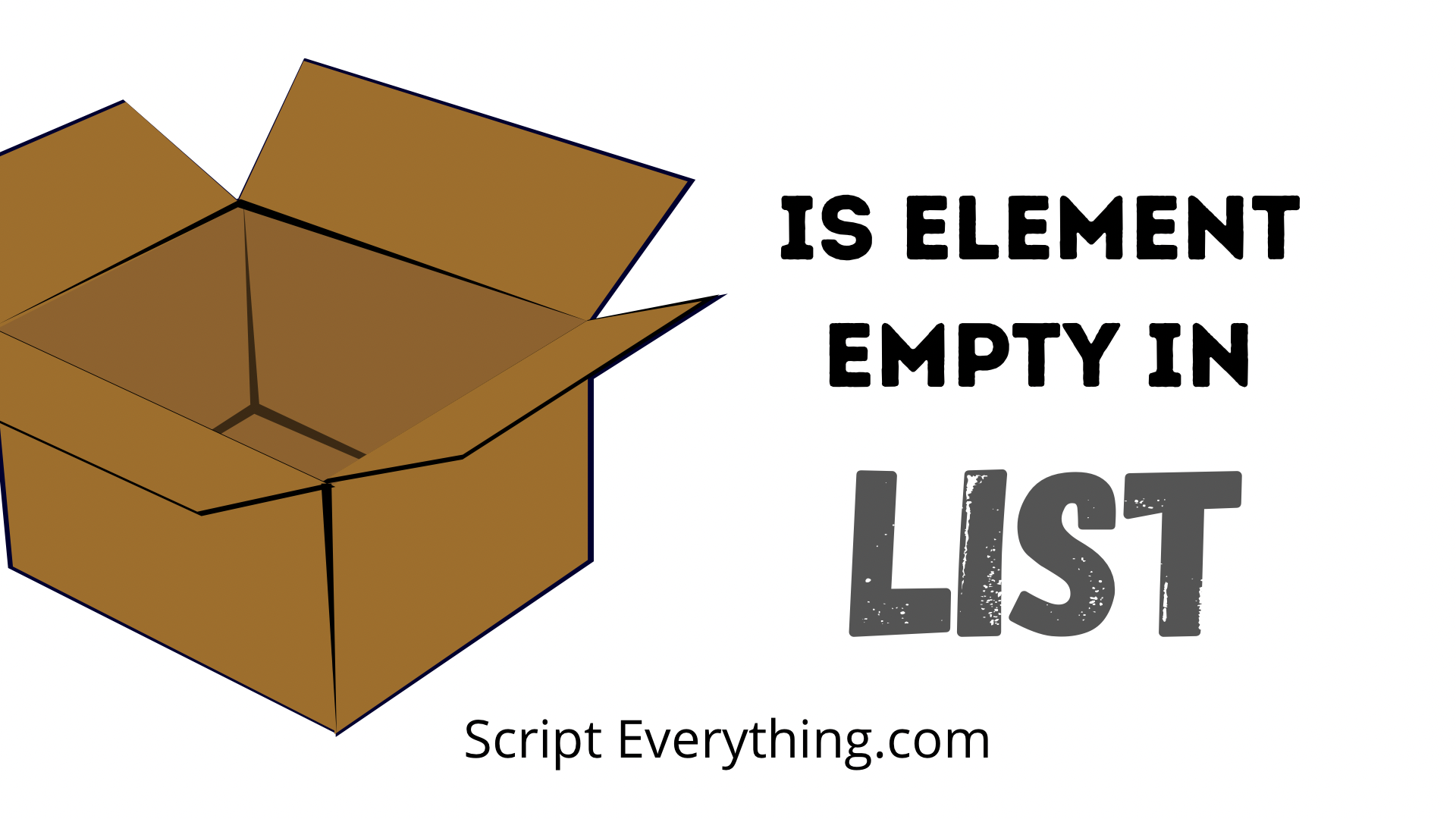# How To Check If Element In List Is Empty In PythonHow do you know if an element in Python is empty? First, you need to define what is meant by the term empty . Does it mean ``` None ``` or an empty string ``` "" ``` or something else? For the examples in this article, I’ll assume that empty means an element is defined as ``` None ``` , however, if you’ve defined empty as something else then you can simply substitute my ``` None ``` references to your definition of an empty element.

To check if an element in a list is empty either perform a direct check by referencing the element using index notation, such as ``` if my_list == None ``` or use a list comprehension to loop through the entire list and perform the necessary check.

Here is an example where you can check if a specific element in your list is empty:

``````>>> e = [None, 2, 3]
>>> if e == None:
...     print("Empty item")
...
Empty item``````

In the above example, the first item in the list is an empty element. Using an if statement I directly reference the first element in the list by using ``` e ``` and compare this directly to what I’m defining as an empty element ``` None ``` . If this is true then I’m printing the output ``` "Empty item" ``` to the REPL.

This is quite a simple way of being able to check if an item is empty, but what if you wanted to know if there was just one empty element in your list?

## Check If List Contains An Empty Element

If your use case is to determine whether a list contains even one empty element and to return ``` True ``` or ``` False ``` based on this condition you can easily do this using one line of code in Python by way of a list comprehension .

Here is an example which I’ll explain further underneath:

``````>>> e = [1, 2, None, 4]
>>> bool([elem for elem in e if elem == None])
True
>>> f = [1, 2, 3, 4]
>>> bool([elem for elem in f if elem == None])
False``````

As demonstrated in the above examples the first list contained an empty element and therefore the result was ``` True ``` , however, the second list did not contain any empty elements and correctly returned ``` False ``` .

The way this code worked was by using a list comprehension with an if statement checking each of the elements in the original list. Through each iteration of the for loop the if statement ensuing checked if there was an empty element, if there was this element was added to the next list being created by the list comprehension.

Once the list comprehension operation was complete the ``` bool() ``` built-in function simply converted the list into a boolean. If the list was empty the boolean returned would be ``` False ``` (which it did in the second case), but if there was at least one item in the list then the boolean would return ``` True ``` (which it did in the first case).

A list comprehension is a great way of being able to perform a task using only one line of Python code.

## Summary

To check if a list element is empty use either a direct index reference to the element by using the syntax ``` list_name[index_number] ``` . If you need to check if a list contains an empty element use the list comprehension code ``` bool([elem for elem in my_list if elem == None]) ``` .

Next, you might want to explore how to add an empty element to a list .

Author of scripteverything.com, Ryan has been dabbling in code since the late '90s when he cut his teeth by exploring VBA in Excel when trying to do something more. Having his eyes opened with the potential of automating repetitive tasks, he expanded to Python and then moved over to scripting languages such as HTML, CSS, Javascript and PHP. When he is not behind a screen, Ryan enjoys a good bush walk with the family during the cooler months, and going with them to the beach during the warmer months.